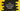# 4 ways in Java to print an inverted right-angled triangle## Java program to print an inverted right-angled triangle:

Let’s learn how to print an inverted right-angled triangle in Java. We will write one program that will take the height of the triangle as an input from the user and print the inverted right-angled triangle using character like *, ^ etc.

I will show you the algorithm and also different ways to print an inverted right-angled triangle.

### Algorithm to print an inverted right-angled triangle:

An inverted right-angled triangle printed with * looks as like below:

``````* * * * * * *
* * * * * *
* * * * *
* * * *
* * *
* *
*``````
• This is a triangle of height 7.
• For the first line, we have to print 7 stars, 6 stars for the second line, 5 stars for the third line etc.

The following algorithm we can use to print the triangle:

• Take the height of the triangle as an input from the user.
• Run one loop for height number of times. This loop will run with a variable from 0 to height - 1. On each iteration, we will increment the value of this variable by 1.
• Run another inner loop to print the body of the triangle. This loop will run for height - outer loop variable value number of times. On each iteration, print the * with a space to its right.
• At the end of each iteration of the outer loop, print a newline to move the outer loop pointer to the next row.

We can use any loop to print this pattern. Let’s learn how to use for loops and while loops:

### Example 1: Java program to print an inverted right-angled triangle using for loops:

Let’s use two for loops to print an inverted right-angled triangle:

``````import java.util.Scanner;

class Main {

public static void main(String[] args) {
int height;
Scanner sc = new Scanner(System.in);

System.out.println("Enter the height of the triangle: ");
height = sc.nextInt();
System.out.println();

for (int i = 0; i < height; i++) {
for (int j = 0; j < height - i; j++) {
System.out.print("*" + " ");
}
System.out.println();
}

}
}``````

In this program,

• The height is taken as an input from the user and stored in the variable height.
• The outer for loop runs from i = 0 to i = height - 1. The inner loop runs from j = 0 to j = height - i. The star is printed in the inner loop.
• At the end of each iteration of the outer loop, one new line is printed.

It will give output as like below:

``````Enter the height of the triangle:
5

* * * * *
* * * *
* * *
* *
* ``````

### Example 2: Java program to print an inverted right-angled triangle using while loops:

We can also use while loops to print the same pattern. Let me change the above program to use while loops:

``````import java.util.Scanner;

class Main {

public static void main(String[] args) {
int height, i = 0, j;
Scanner sc = new Scanner(System.in);

System.out.println("Enter the height of the triangle: ");
height = sc.nextInt();
System.out.println();

while (i < height) {
j = 0;
while (j < height - i) {
System.out.print("*" + " ");
j++;
}
System.out.println();
i++;
}

}
}``````

The variables i and j are initialized before the loops starts and these are incremented at the end of each loop. Other than that, the body of the program is similar to the previous example.

It will give similar result.

### Example 3: Java program to print an inverted right-angled triangle using any character:

Let’s take the character as an input from the user and use that character to print the triangle. We will use another string variable to store that character and use it to print the pattern.

``````import java.util.Scanner;

class Main {

public static void main(String[] args) {
int height, i = 0, j;
String ch;
Scanner sc = new Scanner(System.in);

System.out.println("Enter the height of the triangle: ");
height = sc.nextInt();

System.out.println("Enter the character: ");
ch = sc.next();

System.out.println();

while (i < height) {
j = 0;
while (j < height - i) {
System.out.print(ch + " ");
j++;
}
System.out.println();
i++;
}

}
}``````
• It reads the character and stores it in the string variable ch. This character is used to print the body of the pattern.

It will print output as like below:

``````Enter the height of the triangle:
6
Enter the character:
^

^ ^ ^ ^ ^ ^
^ ^ ^ ^ ^
^ ^ ^ ^
^ ^ ^
^ ^
^

Enter the height of the triangle:
5
Enter the character:
#

# # # # #
# # # #
# # #
# #
# ``````

### Example 4: Java program with a separate method:

We can use a separate method to make the program more reusable. The main method will call the method to print the triangle pattern. It will take the height and the character as its arguments. Let’s see how it works:

``````import java.util.Scanner;

class Main {

private static void printInvertedRightAngledTriangle(int height, String ch) {
int i = 0, j;
while (i < height) {
j = 0;
while (j < height - i) {
System.out.print(ch + " ");
j++;
}
System.out.println();
i++;
}
}

public static void main(String[] args) {
int height;
String ch;
Scanner sc = new Scanner(System.in);

System.out.println("Enter the height of the triangle: ");
height = sc.nextInt();

System.out.println("Enter the character: ");
ch = sc.next();

System.out.println();

printInvertedRightAngledTriangle(height, ch);
}
}``````
• printInvertedRightAngledTriangle method is used to print the inverted right angled triangle. It takes the height and the character as its parameters.
• We are using the same logic to print the triangle in this method. It uses two while loops.

It will print similar output.